# 6+ Metric Unit Conversion Chart Templates

Metric unit conversion charts are so simple to use that anyone between the age of eight to eighty can refer to them to calculate and convert multiple units of the metric system such as length, volume, area, distance, temperature, electric current, pressure and light intensity. The Mertic Conversion Chart Templates are user-friendly and provide accurate conversion units and results which make it convenient for the users to easily make complex calculations in the least amount of time.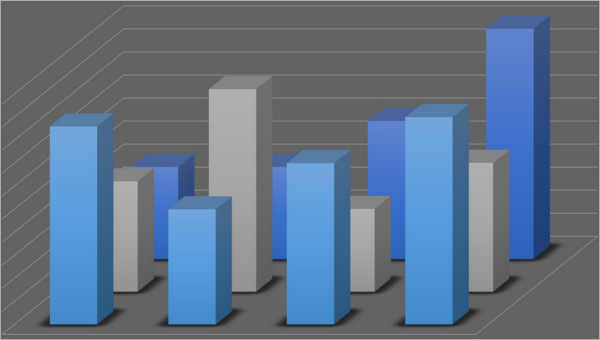## Metric Unit Conversion Chart Worksheet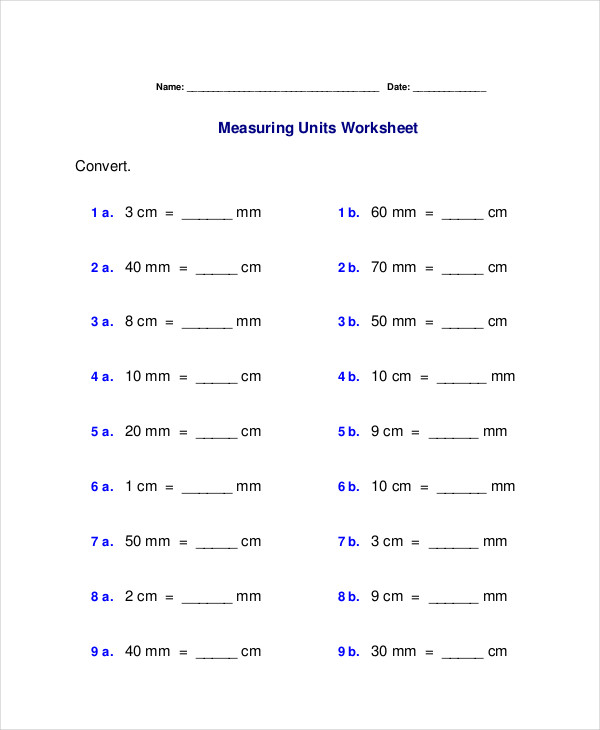homeschoolmath.net

The Metric Unit conversion Chart Templates worksheet can be furnished to students who have just learned the units of Standard or Imperial chart measurements and willing to put their acquired knowledge to testing.

## Basic Metric Unit Conversion Chart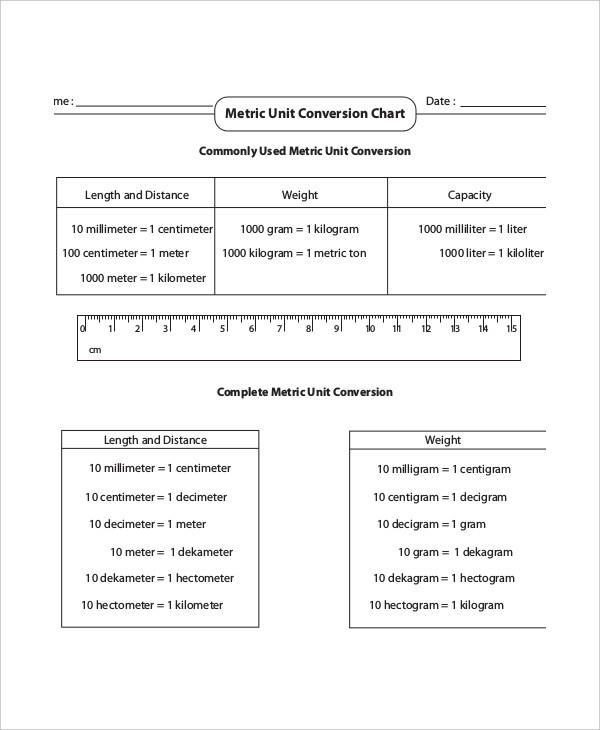mathworksheets4kids.com

You can use the Basic Metric Unit Conversion Chart to make simple calculations and conversions from one unit of measurement to the other such as length, weight area or volume.

## Metric Measurements Conversion Chart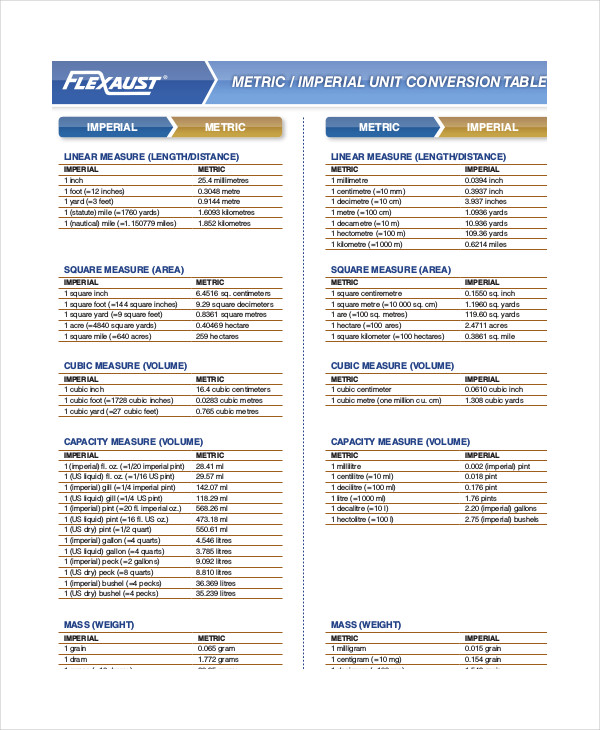flexaust.com

This chart finds it’s usefulness to make simple as well as complex metric unit conversions easy. You can either print it out and save it as a soft copy for reference.

## Unit Conversion Chart Metric & Customary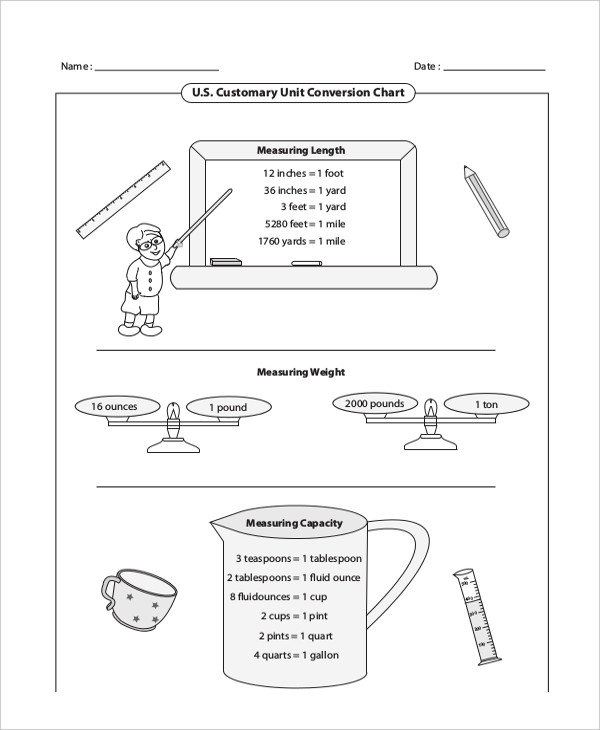mathworksheets4kids.com

This free printable unit conversion chart can assist in converting units between customary (inches, foot, yard, mile, ounce and pound) and metric units (meters, grams, centimeters and kilograms) and vice versa.

## Unit Conversion Chart Metric to Standard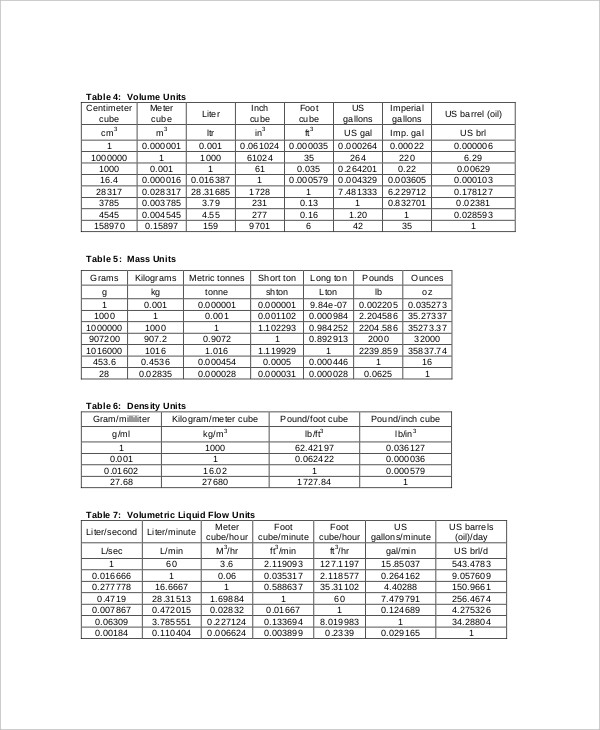isa.org

The Unit conversion chart from metric to the standard system includes the various components of measurement units such as area, speed, currency, volume, length, temperature, pressure, power, distance, time and weight.

## Metric or Imperial Unit Conversion Chart Template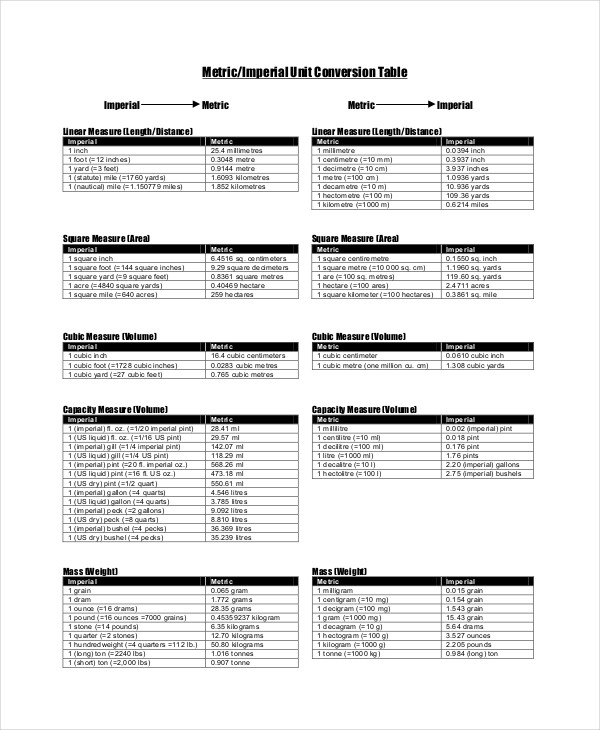hollandpt.com

### > How to use the Metric Unit Conversion Chart?

The Metric Unit conversion chart can be used to make simple as well as complex unit conversions from Imperial, US and Standard metric measures and vice versa. This conversion chart comprises of predetermined values of metric measures as against the other units of measurements which make it absolutely easy for the user to derive the conversion units without having to either understand the complex process or making difficult mathematical calculations. All you have to do is choose the unit of measurement and select the corresponding unit you require to convert it to and fetch the results in minimal given time. You can also see Metric System Conversion Chart Templates.

### > What are the advantages of the Metric Unit Conversion Chart?

The Metric Unit conversion chart is so simple to use that you no longer need to seek the help of scientific calculators or complex mathematics to device values for a given unit of measurement. These charts are available in both the Standard and Imperial types of conversion and can be edited and customized to make them your own. These documents are printable. You also have the option to add some color and definition to the charts to make them visually appealing. Additionally, you can select only the units of measurement you intend to use for your personal or work purpose and leave the rest. You can also see Simple Metric Conversion Chart Templates.

Although the metric system has changed and developed since its inception, its basic concepts have not changed much until this day. Designed for mainly transnational use, this system consists of a basic set of units of measurement, now known as base units which are considered to be the fundamental units before the calculation is made.